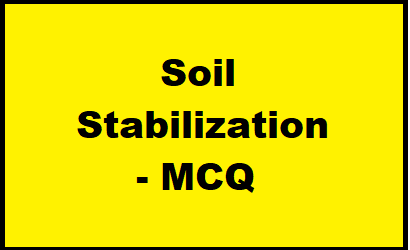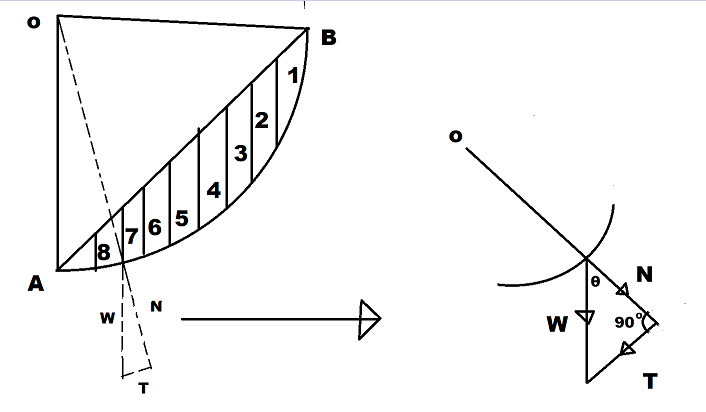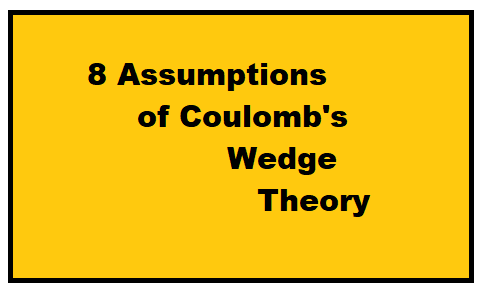Category: soil# Soil Stabilization – MCQ || Soil Engineering

Soil Stabilization - MCQ 1. The aim of soil stabilization is to increase the a) Seepage.b) Bearing capacity.c) Shear strength.d) Both (b) and (c). View Answer d) Both (b) and (c). The aim of soil stabilization is to increase the shear strength and bearing capacity of the soil. 2. In case of stabilization usual proportion … Continue reading Soil Stabilization – MCQ || Soil Engineering# Relationship Between the SPT values with Angle of shearing resistance, Compactness and Relative Density

Relationship Between the SPT values with Angle of shearing resistance, Compactness, and Relative Density The relationship between the standard penetration test(SPT or N) values with angle of shearing resistance(ɸ), compactness, and relative density are as follows: SPT Values (N)CompactnessRelative DensityAngle of shearing resistance(ɸ)0 - 4Very loose0 - 15 <28°4 - 10Loose15 - 3528° - 30°10 … Continue reading Relationship Between the SPT values with Angle of shearing resistance, Compactness and Relative Density# Penetration Tests and Soil Sample Exploration – MCQ

Penetration Tests and Soil Sample Exploration - MCQ 1. The SPT-N value is the number of blows required to drive the sampler through the last a) 15 cmb) 30 cm ✅c) 45 cmd) 50 cm 2. The type of sampler used in standard penetration test is a) Shelby tube samplerb) Piston Samplerc) Split Spoon Sampler … Continue reading Penetration Tests and Soil Sample Exploration – MCQ# Swedish Circle Method For Analyzing The Slope Stability

Swedish Circle Method Swedish circle method for analyzing the slope stability: This method was first introduced by Fellenius (1926) for the analysis of slope stability of C-φ soil. In this method, the soil mass above the assumed slip circle is divided into a number of vertical slices of equal width. The forces between the slices … Continue reading Swedish Circle Method For Analyzing The Slope Stability# 9 Assumptions of Coulomb’s Wedge Theory

9 Assumptions of Coulomb's Wedge Theory Following are the 9 assumptions of Coulomb's wedge theory or Coulomb's wedge failure theory 1. The backfill is dry and cohesionless. 2. The backfill is also homogenous and isotropic. 3. The backfill surface is a plane surface and can be inclined. 4 The back of the wall is vertical … Continue reading 9 Assumptions of Coulomb’s Wedge Theory

# Relation Between Submerged Unit Weight, Specific Gravity, Void Ratio and Unit Weight of Water

Relation Between Submerged Unit Weight, Specific Gravity, Void Ratio and Unit Weight of Water In this article, we shall make a formula or relation between the submerged unit weight(), specific gravity(G), void ratio(e) and unit weight of water/ density of water(). We know, Or, Or, Or, From the definition of submerged unit weight, we have, … Continue reading Relation Between Submerged Unit Weight, Specific Gravity, Void Ratio and Unit Weight of Water

# Typical Values of Permeability for Different Soils

Typical Values of Permeability for Different Soils The permeability or coefficient of permeability can be defined as the rate of flow of water through a unit cross-sectional area of the soil under a unit hydraulic gradient at a temperature of C. Typical values of permeability or coefficient of permeability for different soils are as follows: … Continue reading Typical Values of Permeability for Different Soils